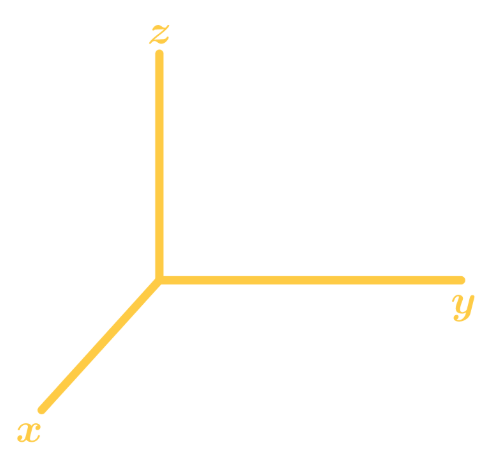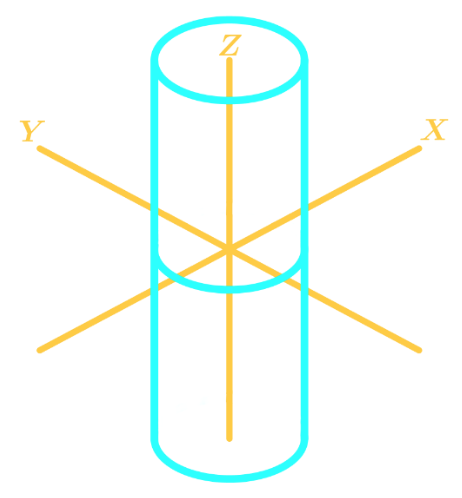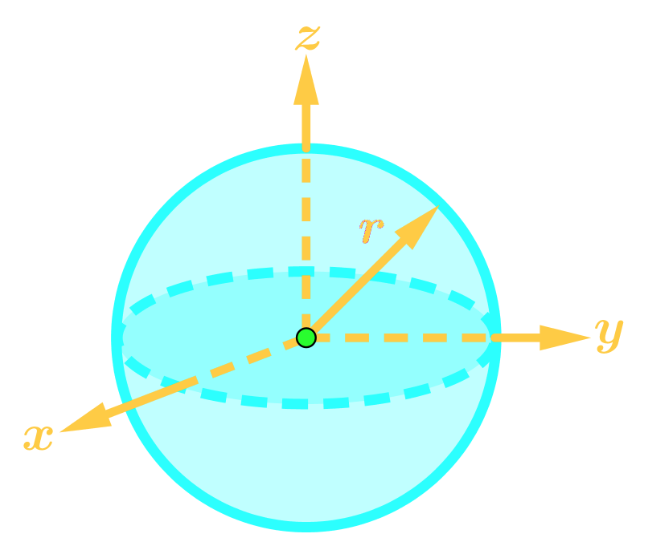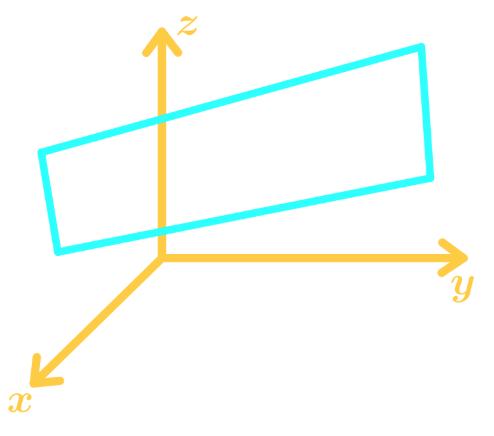# 3-D coordinate system

### 3-D coordinate system

#### Lessons

Notes:

3-D Coordinate System
In the 3-D Coordinate System, also denoted as $\mathbb{R}^3$, we have 3 axis $(x,y,z)$. We draw the axis' in the graph like this:Points are written in the form $P=(x,y,z)$

Planes
The $xy$-plane corresponds to all the points at which $z=0$.
The $xz$-plane corresponds to all the points at which $y=0$.
The $yz$-plane corresponds to all the points at which $x=0$.

These planes are sometimes called "coordinate planes".

Projection of a Point
When a point $P=(x_1,y_1,z_1)$ gets projected onto a plane, then the point will be on the plane.
For example, the projection of point $P=(x_1,y_1,z_1)$ onto the $xy$-plane will become $(x_1,y_1,0)$.

Distance of Two Points To find the distance between two points in $\mathbb{R}^3$, we use the formula:

$D = \sqrt{(x_2-x_1)^2 + (y_2 - y_1)^2 + (z_2 - z_1)^2}$

Where the two points are $P=(x_1,y_1,z_1)$ and $Q=(x_2,y_2,z_2)$.

Other General Equations in 3D
Here are the general types of equations you might see in the 3-D Coordinate system:

1. Cylinder: $(x-a)^2 + (y-b)^2 = r^2$Points are written in the form $P=(x,y,z)$
2. Sphere: $(x-a)^2+(y-b)^2+(z-c)^2=r^2$Points are written in the form $P=(x,y,z)$
3. Plane: $ax+by+cz=d$• Introduction
3-D Coordinate System Overview:
a)
$\mathbb{R}, \mathbb{R}^2, \mathrm{and} \;\mathbb{R}^3$
• Axis in 1D, 2D, and 3D
• Points in 3D
• $xy$-plane, $xz$-plane, and $yz$-plane

b)
Projection & Distance of points
• Projection of a point on a plane
• Distance between two points in 3D
• Knowing and Applying the formula

c)
Graphing & Analyzing Equations
• Graphing the equation $x=3$ in $\mathbb{R}, \mathbb{R}^2, \mathrm{and}\; \mathbb{R}^3$
• Can this equation be graphed in this dimension?
• Other Equations we might see in the course

• 1.
Finding Projection of Points
Find the projection of the point $(-3, 1 ,5)$ onto the $yz$-plane.

• 2.
Finding the Distance of Two Points
Find the distance between $P_1=(1, 0, 4)$ and $P_2=(-2, 3, 5)$.

• 3.
Find the distance between $P_1=(2, -1, -3)$ and $P_2=(4, 0, 1)$.

• 4.
Graphing Equations in Different Dimensions
Graph the equation $y=4$ in 3D.

• 5.
Analyzing Equations in Different Dimensions
Determine whether the equation $(x-2)^2+(y-1)^2=25$ can be graphed in 1D, 2D and 3D.

• 6.
Express a 3D Shape as an Equation
Suppose a sphere is centred at (2,5,3) and the radius of the sphere is 4. Express the sphere as an equation in $\mathbb{R}^3$.

• 7.
Suppose a cylinder is centred at the origin, and the radius of the cylinder is 3 cm. In addition, the cylinder is aligned to the y-axis. Express the cylinder as an equation in $\mathbb{R}^2$.